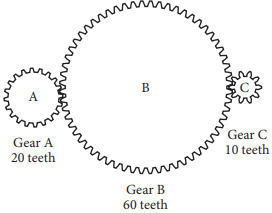mycollegehive
A gear ratio r:s is the ratio of the number of teeth of two ...
SAT-math-test SAT math0A gear ratio r:s is the ratio of the number of teeth of two connected gears. The ratio of the number of revolutions per minute (rpm) of two gear wheels is s:r. In the diagram below, Gear A is turned by a motor. The turning of Gear A causes Gears B and C to turn as well.If Gear A is rotated by the motor at a rate of 100 rpm, what is the number of revolutions per minute for Gear C?

A) 50

B) 110

C) 200

D) 1,000

404 viewsShareFollowUniversity of Lagos Nigeria
29 May 2020University of Lagos Nigeria
02 November 20200Since Gear A has 20 teeth and Gear B has 60 teeth, the gear ratio for Gears A and B is 20:60.

Thus the ratio of the number of revolutions per minute (rpm) for the two gears is 60:20, or 3:1.

That is, when Gear A turns at 3 rpm, Gear B turns at 1 rpm.

Similarly, since Gear B has 60 teeth and Gear C has 10 teeth, the gear ratio for Gears B and C is 60:10, and the ratio of the rpms for the two gears is 10:60.

That is, when Gear B turns at 1 rpm, Gear C turns at 6 rpm.

Therefore, if Gear A turns at 100 rpm, then Gear B turns at 100/3 rpm, and Gear C turns at 100/3 x 6 = 200 rpm.

Ans CShare

### Groups

How to insert math formulas/equations### Related Tags

SAT-math-test

6 followers

476 questionsSAT

3 followers

487 questionsmath

15 followers

1261 questions